# Mechanics LecturePage 1

#### WATCH ALL SLIDES

Slide 1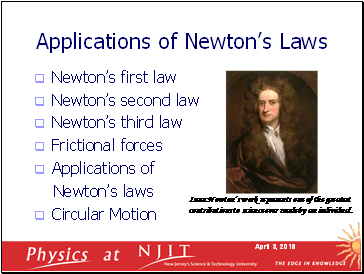## Applications of Newton’s Laws

Newton’s first law

Newton’s second law

Newton’s third law

Frictional forces

Applications of

Newton’s laws

Circular Motion

Isaac Newton’s work represents one of the greatest contributions to science ever made by an individual.

Slide 2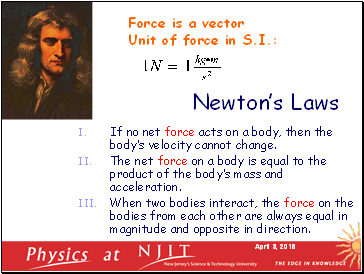Newton’s Laws

If no net force acts on a body, then the body’s velocity cannot change.

The net force on a body is equal to the product of the body’s mass and acceleration.

When two bodies interact, the force on the bodies from each other are always equal in magnitude and opposite in direction.

Force is a vector Unit of force in S.I.:

Slide 3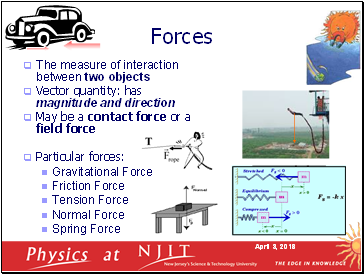## Forces

The measure of interaction between two objects

Vector quantity: has magnitude and direction

May be a contact force or a field force

Particular forces:

Gravitational Force

Friction Force

Tension Force

Normal Force

Spring Force

Slide 4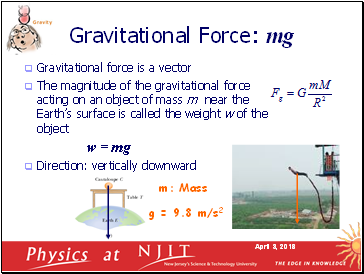Gravitational Force: mg

Gravitational force is a vector

The magnitude of the gravitational force acting on an object of mass m near the Earth’s surface is called the weight w of the object

w = mg

Direction: vertically downward

m: Mass

g = 9.8 m/s2

Slide 5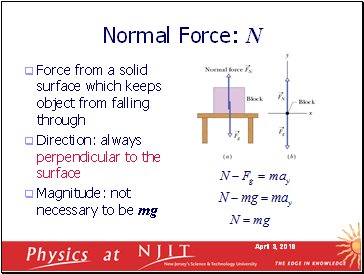Normal Force: N

Force from a solid surface which keeps object from falling through

Direction: always perpendicular to the surface

Magnitude: not necessary to be mg

Slide 6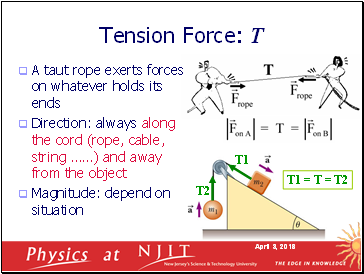Tension Force: T

A taut rope exerts forces on whatever holds its ends

Direction: always along the cord (rope, cable, string ……) and away from the object

Magnitude: depend on situation

T1

T2

T1 = T = T2

Slide 7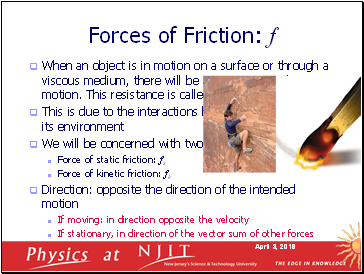When an object is in motion on a surface or through a viscous medium, there will be a resistance to the motion. This resistance is called the force of friction

This is due to the interactions between the object and its environment

We will be concerned with two types of frictional force

Force of static friction: fs

Force of kinetic friction: fk

Direction: opposite the direction of the intended motion

If moving: in direction opposite the velocity

If stationary, in direction of the vector sum of other forces

Forces of Friction: f

Slide 8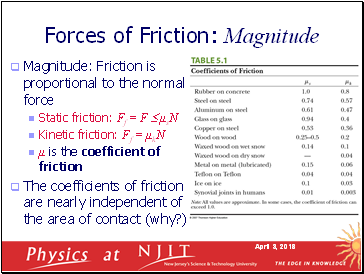Go to page:
1  2  3  4  5